PHP Classes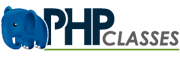# PHP SqlQueryBuilder Class for query building - PHP SQL Query Builder package blog

 Recommend this page to a friend!ReTweet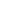All package blogs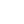PHP SQL Query BuilderPHP SQL Query Builder package blog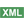PHP SqlQueryBuilder C...Post a comment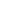See comments (1) Trackbacks (0)

Author:

Updated on: 2019-07-31

Posted on:

Package: PHP SQL Query Builder

A tiny and smart PHP SQL query class for your both complex or basic query needs. It supports usage of joins and complex where clauses, nested queries and etc.By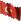ziyahanalbeniz.blogspot.com

<email contact>
A tiny and smart PHP SQL query class for your both complex or basic query needs. It supports usage of joins and complex where clauses, nested queries and etc.
You can write any query what you want using this tiny class. It has been conducted onyle 183 lines of codes and only 5.6KB.

Some of examples you can do with this:
```\$sqlquerybuilder = new SqlQueryBuilder();
\$sqlquerybuilder->select(array(
"col1",
"col2",
"col3"
));
\$sqlquerybuilder->from("table1");
\$sqlquerybuilder->where(array(
array(
"column" => "col1",
"operator" => "=",
"value" => "blabla"
)
));
\$sqlText = \$sqlquerybuilder->build();```
You can use joins: For "inner":
`\$sqlquerybuilder->join("inner","table2",array("table1.id=table2.table1id"));`
For "left":
`\$sqlquerybuilder->join("left","table2",array("table1.id=table2.table1id"));`
Use multi dimensional array for multiple conditionals
```\$sqlquerybuilder->where(array(
array("column"=>"col1","operator"=>"=","value"=>"blabla"),
array("column"=>"col2","operator"=>"=","value"=>"blabla"),
));```
Don't forget it uses "and" logic in where clauses. To use "or" logic in conditions:
```\$sqlquerybuilder->where(array(
array(
"type" => "subset",
"items" => array(
array(
"column" => "job_class_type_name",
"operator" => "=",
"value" => "Published"
) ,
array(
"column" => "job_class_type_name",
"operator" => "=",
"value" => "Approved"
) ,
array(
"column" => "job_class_type_name",
"operator" => "=",
"value" => "Pending"
) ,
array(
"column" => "job_class_type_name",
"operator" => "=",
"value" => "Stopped"
) ,
array(
"column" => "job_class_type_name",
"operator" => "=",
"value" => "Unlisted"
) ,
array(
"column" => "job_class_type_name",
"operator" => "=",
"value" => "Denied"
) ,
array(
"column" => "job_class_type_name",
"operator" => "=",
"value" => "NotEnough"
) ,
)
)
));```
You can also use "in" and "is" operator in where clauses.
```\$sqlquerybuilder->where(array(
array("column"=>"col1","operator"=>"in","value"=>"(1,2,3)"),
array("column"=>"col2","operator"=>"is","value"=>"null")
));```
For grouping :
`\$sqlquerybuilder->groupBy(array("col1"));`
Ordering:
```\$sqlquerybuilder->orderBy(
array(
array("field"=>"col1","dir"=>"desc"),
array("field"=>"col2","dir"=>"desc")
)
);```
To put limits:
`\$sqlquerybuilder->limits(array("start"=>0,"limit"=>10));`
We have seen writing line by line so far. Certainly it supports chaining query:
```\$sqlText = \$sqlquerybuilder->select(array(
"col1",
"col2",
"col3"
))->from("table1")->join("inner", "table2", array(
"table2.table1id=table1.id"
))->where(array(
"column" =>"col1",
"operator" =>"in",
"value" =>"(1,2,3)"
) , array(
"column" =>"col2",
"operator" =>"is",
"value" =>"null"
))->groupBy(array(
"col1"
))->orderby(array(
array(
"field" => "col1",
"dir" => "desc"
)
))->limit(array(
"start" => 0,
"limit" => 10
))->build()```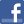Facebook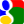Gmail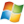Hotmail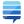StackOverflow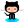GitHub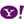YahooPost a commentSee comments (1) Trackbacks (0)All package blogsPHP SQL Query BuilderPHP SQL Query Builder package blogPHP SqlQueryBuilder C...Advertise on this siteSite mapNewsletterStatisticsSite tipsPrivacy policyContact